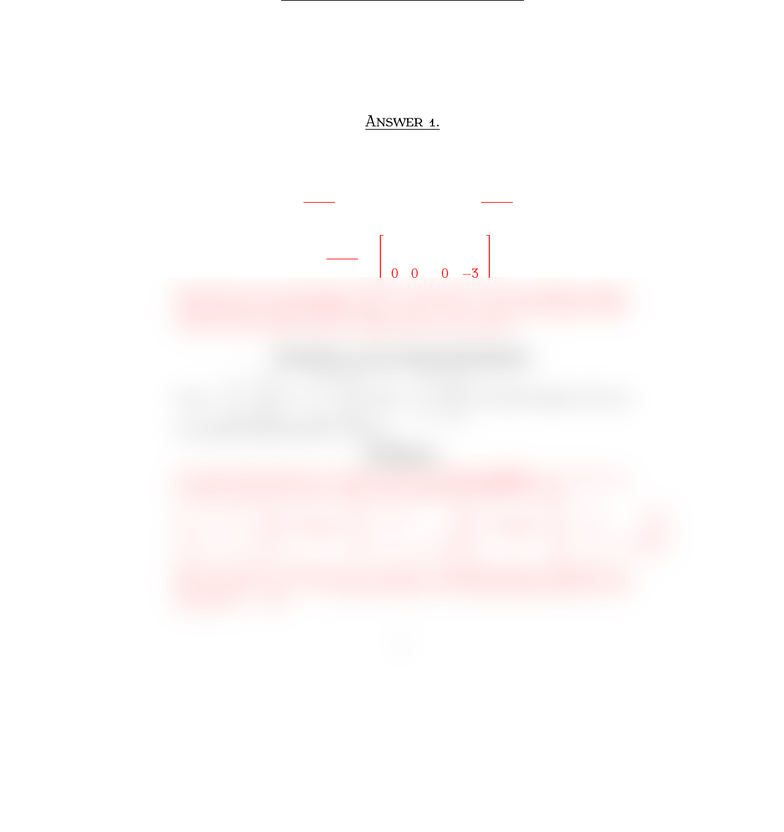Study Guides (400,000)
US (230,000)
BC (3,000)
MATH (90)
All (80)
Quiz

# Quiz2mt210Sample2AnsExam

Department
Mathematics
Course Code
MATH 2211
Professor
All
Study Guide
Quiz

This preview shows half of the first page. to view the full 1 pages of the document.MT Q  S 
İ S. Y
F , 
Surname, Name:
Q . §.V E
Is b=
1
1
3
a linear combination of the vectors a1=
0
1
1
,a2=
1
1
2
and a3=
1
1
2
?
A .
To answer this question, we need to solve the vector equation x1a1+x2a2+
x3a3=b. In other words, we need to reduce the augmented matrix [a1a2a3b].
0 1 1 1
1 1 11
1 2 23
R1R2
//
1 1 11
0 1 1 1
1 2 23
R1+R3R3
//
1 1 11
0 1 1 1
0 1 12
R2+R3R3
//
1 1 11
0 1 1 1
0 0 0 3
Note that the last augmented matrix corresponds to an inconsistent system.
Therefore, the vector equation x1a1+x2a2+x3a3=bhas no solution which
means that bis not a linear combination of a1,a2and a3.
Q . §. V E
Let v1=
1
0
2
,v2=
3
1
8
, and y=
h
5
3
. For what value(s) of his y
in the plane generated by v1and v2?
A .
To answer this question, we need to solve the vector equation x1v1+x2v2=y.
In other words, we need to reduce the augmented matrix [v1v2y].
13h
0 1 5
2 8 3
2R1+R3R3
//
13h
0 1 5
0 2 3 + 2h
2R2+R3R3
//
13h
0 1 5
0 0 7 + 2h
Since we want ySpan{v1,v2}, we need a consistent system. Therefore, we
have to have 7 + 2h= 0. In other words, yis in the plane generated by v1and
v2when h=7/2.
1